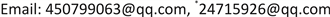﻿ 基于均差估计二变量Meta-分析的未知不相等方差模型 The Unknown Unequal Variance Model of Bivariate Meta-Analysis Based on the Difference Mean

Operations Research and Fuzziology
Vol. 09  No. 01 ( 2019 ), Article ID: 28809 , 8 pages
10.12677/ORF.2019.91009

The Unknown Unequal Variance Model of Bivariate Meta-Analysis Based on the Difference Mean

Pengfei Guo1,2,3, Gang Li4*, Xiangru Sun5

1College of Computational Science, Zhongkai University of Agriculture and Engineering, Guangzhou Guangdong

2Intelligent Agriculture Engineering Research Center of Guangdong Higher Education Institute, Zhongkai University of Agriculture and Engineering, Guangzhou Guangdong

3Guangdong Province Key Laboratory of Waterfowl Healthy Breeding, Zhongkai University of Agriculture and Engineering, Guangzhou Guangdong

4Guangzhou E-Government Center, Guangzhou Guangdong

5Institute of Reproductive Medicine, Panyu Hexian Memorial Hospital of Guangzhou, Guangzhou GuangdongReceived: Jan. 15th, 2019; accepted: Feb. 5th, 2019; published: Feb. 12th, 2019ABSTRACT

This paper introduces the unknown unequal variance model of bivariate meta-analysis based on the difference mean; we obtain the maximum likelihood estimator of the difference mean effect and unknown variances. Then we find the combining overall difference mean effect ${D}_{overall}$ by the fixed-effect model of bivariate Meta-analysis based on the difference mean. Moreover, we obtain the corresponding covariance matrix and the $\left(1-\alpha \right)100%$ confidence interval for the overall difference mean effect ${D}_{overall}$ .

Keywords:Meta-Analysis, The Unknown Unequal Variance Model, Maximum Likelihood Estimate1仲恺农业工程学院，计算科学学院，广东 广州

2仲恺农业工程学院，广东省高校智慧农业工程研究中心，广东 广州

3仲恺农业工程学院，广东省水禽健康养殖重点实验室，广东 广州

4广州市电子政务服务中心，广东 广州

5番禺何贤纪念医院，生殖医学科，广东 广州1. 引言

Meta-分析回答了一个非常广为接受的问题和设计。即什么是研究的目标？在一个广泛的整体中研究的目标是否能够得到确认？什么是研究结果的可操作定义：试验样本组还是研究对象的总体？什么样类型的设计在搜索过程中是需要包括的？只有检验研究假设的随机试验可以作为纳入文献？非试验的研究是否可以作为纳入文献？上述这些问题的答案影响了一般综述的方法，统计推断的模式和研究结果的解释。

Meta-分析是一种对同一问题的不同研究进行定量合并的一种方法。针对具体问题的Meta-分析及其统计方法研究已持续了一个世纪之久。最早是在1904年Karl Pearson针对伤寒疫苗效果进行了合并研究  ；但是在过去的四十年中，人们逐渐意识到医学实验和临床操作需要基于整体的相关性和可靠证据，Meta-分析的影响得到极大的扩展  。

2. 问题描述

${D}_{i}=\left(\begin{array}{c}{D}_{i1}\\ {D}_{i2}\end{array}\right)~N\left(\left(\begin{array}{c}{\mu }_{1}\\ {\mu }_{2}\end{array}\right),\left(\begin{array}{cc}{\sigma }_{C}^{2}{w}_{i1}^{C}+{\sigma }_{T}^{2}{w}_{i1}^{T}& 0\\ 0& {\tau }_{C}^{2}{w}_{i2}^{C}+{\tau }_{T}^{2}{w}_{i2}^{T}\end{array}\right)\right)$

3. 均值效应量的极大似然估计

$\begin{array}{c}L\left(\mu ,{D}_{i},{S}_{i}\right)=L\left({\mu }^{C},{\stackrel{¯}{X}}_{i}^{C},{S}_{i}^{C}\right)L\left({\mu }^{T},{\stackrel{¯}{X}}_{i}^{T},{S}_{i}^{T}\right)\\ =\underset{i=1}{\overset{k}{\prod }}\frac{1}{2\text{π}det{\left({S}_{i}^{C}\right)}^{\frac{1}{2}}}\mathrm{exp}\left\{-\frac{{\left({\stackrel{¯}{X}}_{i}^{C}-{\mu }^{C}\right)}^{\text{T}}{\left({S}_{i}^{C}\right)}^{-1}\left({\stackrel{¯}{X}}_{i}^{C}-{\mu }^{C}\right)}{2}\right\}\\ \text{\hspace{0.17em}}\text{\hspace{0.17em}}\cdot \underset{i=1}{\overset{k}{\prod }}\frac{1}{2\text{π}det{\left({S}_{i}^{T}\right)}^{\frac{1}{2}}}\mathrm{exp}\left\{-\frac{{\left({\stackrel{¯}{X}}_{i}^{T}-{\mu }^{T}\right)}^{\text{T}}{\left({S}_{i}^{T}\right)}^{-1}\left({\stackrel{¯}{X}}_{i}^{T}-{\mu }^{T}\right)}{2}\right\}\end{array}$

$L\left({\mu }^{C},{\stackrel{¯}{X}}_{i}^{C},{S}_{i}^{C}\right)=-k\mathrm{ln}\left(\text{2π}\right)-\frac{1}{2}\underset{i=1}{\overset{k}{\sum }}\mathrm{ln}\left[det\left({S}_{i}^{C}\right)\right]-\frac{1}{2}\underset{i=1}{\overset{k}{\sum }}\frac{{\left({\stackrel{¯}{X}}_{i}^{C}-{\mu }^{C}\right)}^{\text{T}}{\left({S}_{i}^{C}\right)}^{-1}\left({\stackrel{¯}{X}}_{i}^{C}-{\mu }^{C}\right)}{2},$

$L\left({\mu }^{T},{\stackrel{¯}{X}}_{i}^{T},{S}_{i}^{T}\right)=-k\mathrm{ln}\left(\text{2π}\right)-\frac{1}{2}\underset{i=1}{\overset{k}{\sum }}\mathrm{ln}\left[det\left({S}_{i}^{T}\right)\right]-\frac{1}{2}\underset{i=1}{\overset{k}{\sum }}\frac{{\left({\stackrel{¯}{X}}_{i}^{T}-{\mu }^{T}\right)}^{\text{T}}{\left({S}_{i}^{T}\right)}^{-1}\left({\stackrel{¯}{X}}_{i}^{T}-{\mu }^{T}\right)}{2}.$

1) $\mu$ 的极大似然估计量的分量为：

${\stackrel{^}{\mu }}_{1}={\stackrel{^}{\mu }}_{1}^{T}-{\stackrel{^}{\mu }}_{1}^{C}=\frac{\underset{i=1}{\overset{k}{\sum }}\frac{{\stackrel{¯}{X}}_{i1}^{T}}{{w}_{i1}^{T}}}{\underset{i=1}{\overset{k}{\sum }}\frac{1}{{w}_{i1}^{T}}}-\frac{\underset{i=1}{\overset{k}{\sum }}\frac{{\stackrel{¯}{X}}_{i1}^{C}}{{w}_{i1}^{C}}}{\underset{i=1}{\overset{k}{\sum }}\frac{1}{{w}_{i1}^{C}}},\text{\hspace{0.17em}}\text{\hspace{0.17em}}{\stackrel{^}{\mu }}_{2}={\stackrel{^}{\mu }}_{2}^{T}-{\stackrel{^}{\mu }}_{2}^{C}=\frac{\underset{i=1}{\overset{k}{\sum }}\frac{{\stackrel{¯}{X}}_{i2}^{T}}{{w}_{i2}^{T}}}{\underset{i=1}{\overset{k}{\sum }}\frac{1}{{w}_{i2}^{T}}}-\frac{\underset{i=1}{\overset{k}{\sum }}\frac{{\stackrel{¯}{X}}_{i2}^{C}}{{w}_{i2}^{C}}}{\underset{i=1}{\overset{k}{\sum }}\frac{1}{{w}_{i2}^{C}}}.$

2) ${\sigma }^{2}$${\tau }^{2}$ 的极大似然估计量分别为：

${\stackrel{^}{\sigma }}_{CML}^{2}=\frac{1}{k}\underset{i=1}{\overset{k}{\sum }}\frac{{\left({\stackrel{¯}{X}}_{i1}^{C}-{\stackrel{^}{\mu }}_{1}^{C}\right)}^{2}}{{w}_{i1}^{C}},\text{\hspace{0.17em}}{\stackrel{^}{\tau }}_{CML}^{2}=\frac{1}{k}\underset{i=1}{\overset{k}{\sum }}\frac{{\left({\stackrel{¯}{X}}_{i2}^{C}-{\stackrel{^}{\mu }}_{1}^{C}\right)}^{2}}{{w}_{i2}^{C}};$

${\stackrel{^}{\sigma }}_{TML}^{2}=\frac{1}{k}\underset{i=1}{\overset{k}{\sum }}\frac{{\left({\stackrel{¯}{X}}_{i1}^{T}-{\stackrel{^}{\mu }}_{1}^{T}\right)}^{2}}{{w}_{i1}^{C}},\text{\hspace{0.17em}}{\stackrel{^}{\tau }}_{TML}^{2}=\frac{1}{k}\underset{i=1}{\overset{k}{\sum }}\frac{{\left({\stackrel{¯}{X}}_{i2}^{T}-{\stackrel{^}{\mu }}_{1}^{T}\right)}^{2}}{{w}_{i2}^{T}}.$

${\stackrel{^}{\mu }}_{1}={\stackrel{^}{\mu }}_{1}^{T}-{\stackrel{^}{\mu }}_{1}^{C}=\frac{\underset{i=1}{\overset{k}{\sum }}\frac{{\stackrel{¯}{X}}_{i1}^{T}}{{w}_{i1}^{T}}}{\underset{i=1}{\overset{k}{\sum }}\frac{1}{{w}_{i1}^{T}}}-\frac{\underset{i=1}{\overset{k}{\sum }}\frac{{\stackrel{¯}{X}}_{i1}^{C}}{{w}_{i1}^{C}}}{\underset{i=1}{\overset{k}{\sum }}\frac{1}{{w}_{i1}^{C}}},\text{\hspace{0.17em}}\text{\hspace{0.17em}}{\stackrel{^}{\mu }}_{2}={\stackrel{^}{\mu }}_{2}^{T}-{\stackrel{^}{\mu }}_{2}^{C}=\frac{\underset{i=1}{\overset{k}{\sum }}\frac{{\stackrel{¯}{X}}_{i2}^{T}}{{w}_{i2}^{T}}}{\underset{i=1}{\overset{k}{\sum }}\frac{1}{{w}_{i2}^{T}}}-\frac{\underset{i=1}{\overset{k}{\sum }}\frac{{\stackrel{¯}{X}}_{i2}^{C}}{{w}_{i2}^{C}}}{\underset{i=1}{\overset{k}{\sum }}\frac{1}{{w}_{i2}^{C}}}.$

${\stackrel{^}{\mu }}_{ML}$ 对于 $\mu$ 是无偏的。

$\begin{array}{c}E\left({\stackrel{^}{\mu }}_{1}^{T}-{\stackrel{^}{\mu }}_{1}^{C}\right)=E\left\{\frac{\underset{i=1}{\overset{k}{\sum }}\frac{{\stackrel{¯}{X}}_{i1}^{T}}{{w}_{i1}^{T}}}{\underset{i=1}{\overset{k}{\sum }}\frac{1}{{w}_{i1}^{T}}}-\frac{\underset{i=1}{\overset{k}{\sum }}\frac{{\stackrel{¯}{X}}_{i1}^{C}}{{w}_{i1}^{C}}}{\underset{i=1}{\overset{k}{\sum }}\frac{1}{{w}_{i1}^{C}}}\right\}=E\left\{\frac{\underset{i=1}{\overset{k}{\sum }}\frac{{\stackrel{¯}{X}}_{i1}^{T}}{{w}_{i1}^{T}}}{\underset{i=1}{\overset{k}{\sum }}\frac{1}{{w}_{i1}^{T}}}\right\}-E\left\{\frac{\underset{i=1}{\overset{k}{\sum }}\frac{{\stackrel{¯}{X}}_{i1}^{C}}{{w}_{i1}^{C}}}{\underset{i=1}{\overset{k}{\sum }}\frac{1}{{w}_{i1}^{C}}}\right\}\\ =\frac{\underset{i=1}{\overset{k}{\sum }}\frac{E\left\{{\stackrel{¯}{X}}_{i1}^{T}\right\}}{{w}_{i1}^{T}}}{\underset{i=1}{\overset{k}{\sum }}\frac{1}{{w}_{i1}^{T}}}-\frac{\underset{i=1}{\overset{k}{\sum }}\frac{E\left\{{\stackrel{¯}{X}}_{i1}^{C}\right\}}{{w}_{i1}^{C}}}{\underset{i=1}{\overset{k}{\sum }}\frac{1}{{w}_{i1}^{C}}}=\frac{\underset{i=1}{\overset{k}{\sum }}\frac{{\mu }_{1}^{T}}{{w}_{i1}^{T}}}{\underset{i=1}{\overset{k}{\sum }}\frac{1}{{w}_{i1}^{T}}}-\frac{\underset{i=1}{\overset{k}{\sum }}\frac{{\mu }_{1}^{C}}{{w}_{i1}^{C}}}{\underset{i=1}{\overset{k}{\sum }}\frac{1}{{w}_{i1}^{C}}}\\ ={\mu }_{1}^{T}-{\mu }_{1}^{C}\end{array}$

$\begin{array}{c}E\left({\stackrel{^}{\mu }}_{2}^{T}-{\stackrel{^}{\mu }}_{2}^{C}\right)=E\left\{\frac{\underset{i=1}{\overset{k}{\sum }}\frac{{\stackrel{¯}{X}}_{i2}^{T}}{{w}_{i2}^{T}}}{\underset{i=1}{\overset{k}{\sum }}\frac{1}{{w}_{i2}^{T}}}-\frac{\underset{i=1}{\overset{k}{\sum }}\frac{{\stackrel{¯}{X}}_{i2}^{C}}{{w}_{i1}^{C}}}{\underset{i=1}{\overset{k}{\sum }}\frac{1}{{w}_{i2}^{C}}}\right\}=E\left\{\frac{\underset{i=1}{\overset{k}{\sum }}\frac{{\stackrel{¯}{X}}_{i2}^{T}}{{w}_{i2}^{T}}}{\underset{i=1}{\overset{k}{\sum }}\frac{1}{{w}_{i2}^{T}}}\right\}-E\left\{\frac{\underset{i=1}{\overset{k}{\sum }}\frac{{\stackrel{¯}{X}}_{i2}^{C}}{{w}_{i2}^{C}}}{\underset{i=1}{\overset{k}{\sum }}\frac{1}{{w}_{i2}^{C}}}\right\}\\ =\frac{\underset{i=1}{\overset{k}{\sum }}\frac{E\left\{{\stackrel{¯}{X}}_{i2}^{T}\right\}}{{w}_{i2}^{T}}}{\underset{i=1}{\overset{k}{\sum }}\frac{1}{{w}_{i2}^{T}}}-\frac{\underset{i=1}{\overset{k}{\sum }}\frac{E\left\{{\stackrel{¯}{X}}_{i2}^{C}\right\}}{{w}_{i2}^{C}}}{\underset{i=1}{\overset{k}{\sum }}\frac{1}{{w}_{i2}^{C}}}=\frac{\underset{i=1}{\overset{k}{\sum }}\frac{{\mu }_{1}^{T}}{{w}_{i2}^{T}}}{\underset{i=1}{\overset{k}{\sum }}\frac{1}{{w}_{i2}^{T}}}-\frac{\underset{i=1}{\overset{k}{\sum }}\frac{{\mu }_{1}^{C}}{{w}_{i2}^{C}}}{\underset{i=1}{\overset{k}{\sum }}\frac{1}{{w}_{i2}^{C}}}\\ ={\mu }_{2}^{T}-{\mu }_{2}^{C}\end{array}$

4. 均值效应量极大似然估计量的协方差矩阵

${\stackrel{^}{\mu }}_{1}={\stackrel{^}{\mu }}_{1}^{T}-{\stackrel{^}{\mu }}_{1}^{C}=\frac{\underset{i=1}{\overset{k}{\sum }}\frac{{\stackrel{¯}{X}}_{i1}^{T}}{{w}_{i1}^{T}}}{\underset{i=1}{\overset{k}{\sum }}\frac{1}{{w}_{i1}^{T}}}-\frac{\underset{i=1}{\overset{k}{\sum }}\frac{{\stackrel{¯}{X}}_{i1}^{C}}{{w}_{i1}^{C}}}{\underset{i=1}{\overset{k}{\sum }}\frac{1}{{w}_{i1}^{C}}},\text{\hspace{0.17em}}\text{\hspace{0.17em}}{\stackrel{^}{\mu }}_{2}={\stackrel{^}{\mu }}_{2}^{T}-{\stackrel{^}{\mu }}_{2}^{C}=\frac{\underset{i=1}{\overset{k}{\sum }}\frac{{\stackrel{¯}{X}}_{i2}^{T}}{{w}_{i2}^{T}}}{\underset{i=1}{\overset{k}{\sum }}\frac{1}{{w}_{i2}^{T}}}-\frac{\underset{i=1}{\overset{k}{\sum }}\frac{{\stackrel{¯}{X}}_{i2}^{C}}{{w}_{i2}^{C}}}{\underset{i=1}{\overset{k}{\sum }}\frac{1}{{w}_{i2}^{C}}}.$

${\stackrel{^}{\mu }}_{ML}$ 所对应的协方差矩阵 $Var\left({\stackrel{^}{\mu }}_{ML}\right)$ 为：

$Var\left({\stackrel{^}{\mu }}_{ML}\right)=\left(\begin{array}{cc}\frac{{\sigma }_{C}^{2}}{\underset{i=1}{\overset{k}{\sum }}\frac{1}{{w}_{i1}^{C}}}+\frac{{\sigma }_{T}^{2}}{\underset{i=1}{\overset{k}{\sum }}\frac{1}{{w}_{i1}^{T}}}& 0\\ 0& \frac{{\tau }_{C}^{2}}{\underset{i=1}{\overset{k}{\sum }}\frac{1}{{w}_{i2}^{C}}}+\frac{{\tau }_{T}^{2}}{\underset{i=1}{\overset{k}{\sum }}\frac{1}{{w}_{i2}^{T}}}\end{array}\right)$

${\stackrel{^}{\mu }}_{1}={\stackrel{^}{\mu }}_{1}^{T}-{\stackrel{^}{\mu }}_{1}^{C}=\frac{\underset{i=1}{\overset{k}{\sum }}\frac{{\stackrel{¯}{X}}_{i1}^{T}}{{w}_{i1}^{T}}}{\underset{i=1}{\overset{k}{\sum }}\frac{1}{{w}_{i1}^{T}}}-\frac{\underset{i=1}{\overset{k}{\sum }}\frac{{\stackrel{¯}{X}}_{i1}^{C}}{{w}_{i1}^{C}}}{\underset{i=1}{\overset{k}{\sum }}\frac{1}{{w}_{i1}^{C}}},\text{\hspace{0.17em}}\text{\hspace{0.17em}}{\stackrel{^}{\mu }}_{2}={\stackrel{^}{\mu }}_{2}^{T}-{\stackrel{^}{\mu }}_{2}^{C}=\frac{\underset{i=1}{\overset{k}{\sum }}\frac{{\stackrel{¯}{X}}_{i2}^{T}}{{w}_{i2}^{T}}}{\underset{i=1}{\overset{k}{\sum }}\frac{1}{{w}_{i2}^{T}}}-\frac{\underset{i=1}{\overset{k}{\sum }}\frac{{\stackrel{¯}{X}}_{i2}^{C}}{{w}_{i2}^{C}}}{\underset{i=1}{\overset{k}{\sum }}\frac{1}{{w}_{i2}^{C}}}.$

${\stackrel{^}{\mu }}_{ML}$ 对于 $\mu$ 是一致的。

5. 整体均值合并统计量的估计

1) 对于均值合并统计量，每个研究均差的权重为：

${\nu }_{i}={\left(\underset{i=1}{\overset{k}{\sum }}{\stackrel{^}{S}}_{iML}^{-1}\right)}^{-1}{\stackrel{^}{S}}_{iML}^{-1}=\left(\begin{array}{cc}\frac{\frac{1}{{\stackrel{^}{\sigma }}_{CML}^{2}{w}_{i1}^{C}+{\stackrel{^}{\sigma }}_{TML}^{2}{w}_{i1}^{T}}}{\underset{i=1}{\overset{k}{\sum }}\frac{1}{{\stackrel{^}{\sigma }}_{CML}^{2}{w}_{i1}^{C}+{\stackrel{^}{\sigma }}_{TML}^{2}{w}_{i1}^{T}}}& 0\\ 0& \frac{\frac{1}{{\stackrel{^}{\tau }}_{CML}^{2}{w}_{i2}^{C}+{\stackrel{^}{\tau }}_{TML}^{2}{w}_{i2}^{T}}}{\underset{i=1}{\overset{k}{\sum }}\frac{1}{{\stackrel{^}{\tau }}_{CML}^{2}{w}_{i2}^{C}+{\stackrel{^}{\tau }}_{TML}^{2}{w}_{i2}^{T}}}\end{array}\right)$

2) 整体均值合并统计量的估计量为：

${D}_{\text{overall}}=\underset{i=1}{\overset{k}{\sum }}{D}_{i}{\nu }_{i}=\left(\begin{array}{c}\frac{\frac{{\stackrel{¯}{X}}_{i1}^{T}-{\stackrel{¯}{X}}_{i1}^{C}}{{\stackrel{^}{\sigma }}_{CML}^{2}{w}_{i1}^{C}+{\stackrel{^}{\sigma }}_{TML}^{2}{w}_{i1}^{T}}}{\underset{i=1}{\overset{k}{\sum }}\frac{1}{{\stackrel{^}{\sigma }}_{CML}^{2}{w}_{i1}^{C}+{\stackrel{^}{\sigma }}_{TML}^{2}{w}_{i1}^{T}}}\\ \frac{\frac{{\stackrel{¯}{X}}_{i1}^{T}-{\stackrel{¯}{X}}_{i1}^{C}}{{\stackrel{^}{\sigma }}_{CML}^{2}{w}_{i1}^{C}+{\stackrel{^}{\sigma }}_{TML}^{2}{w}_{i1}^{T}}}{\underset{i=1}{\overset{k}{\sum }}\frac{1}{{\stackrel{^}{\sigma }}_{CML}^{2}{w}_{i1}^{C}+{\stackrel{^}{\sigma }}_{TML}^{2}{w}_{i1}^{T}}}\end{array}\right)$${\stackrel{^}{\mu }}_{1}={\stackrel{^}{\mu }}_{1}^{T}-{\stackrel{^}{\mu }}_{1}^{C}=\frac{\underset{i=1}{\overset{k}{\sum }}\frac{{\stackrel{¯}{X}}_{i1}^{T}}{{w}_{i1}^{T}}}{\underset{i=1}{\overset{k}{\sum }}\frac{1}{{w}_{i1}^{T}}}-\frac{\underset{i=1}{\overset{k}{\sum }}\frac{{\stackrel{¯}{X}}_{i1}^{C}}{{w}_{i1}^{C}}}{\underset{i=1}{\overset{k}{\sum }}\frac{1}{{w}_{i1}^{C}}},\text{\hspace{0.17em}}\text{\hspace{0.17em}}{\stackrel{^}{\mu }}_{2}={\stackrel{^}{\mu }}_{2}^{T}-{\stackrel{^}{\mu }}_{2}^{C}=\frac{\underset{i=1}{\overset{k}{\sum }}\frac{{\stackrel{¯}{X}}_{i2}^{T}}{{w}_{i2}^{T}}}{\underset{i=1}{\overset{k}{\sum }}\frac{1}{{w}_{i2}^{T}}}-\frac{\underset{i=1}{\overset{k}{\sum }}\frac{{\stackrel{¯}{X}}_{i2}^{C}}{{w}_{i2}^{C}}}{\underset{i=1}{\overset{k}{\sum }}\frac{1}{{w}_{i2}^{C}}}.$

6. 结束语

The Unknown Unequal Variance Model of Bivariate Meta-Analysis Based on the Difference Mean[J]. 运筹与模糊学, 2019, 09(01): 72-79. https://doi.org/10.12677/ORF.2019.91009

1. 1. Pearson, K. (1904) Report on Certain Enteric Fever Inoculation Statistics. British Medical Journal, 3, 1243-1246.

2. 2. Lee, W.L., Bausell, R.B. and Berman, B.M. (2001) The Growth of Health-Related Meta-Analyses Published from 1980 to 2000. Evaluation and the Health Professions, 24, 327-335. https://doi.org/10.1177/01632780122034948

3. 3. Stijnen, T. (1999) Tutorial in Biostatistics. Meta-Analysis: Formulating, Evaluating, Combining, and Reporting by S-L. Normand. Statistics in Medicine, 18, 321-359.

4. 4. Raudenbush, S.W., Becker, B.J. and Kalaian, H. (1988) Modeling Multivariate Effect Sizes. Psychological Bulletin, 103, 111-120. https://doi.org/10.1037/0033-2909.103.1.111

5. 5. van Houwelingen, H.C., Zwinderman, K.H. and Stijnen, T. (1993) A Bivariate Approach to Meta-Analysis. Statistics in Medicine, 12, 2273-2284. https://doi.org/10.1002/sim.4780122405

6. 6. van Houwelingen, H.C., Arends, L.R. and Stijnen, T. (2002) Advanced Methods in Meta-Analysis: Multivariate Approach and Metaregression. Statistics in Medicine, 21, 589-624. https://doi.org/10.1002/sim.1040

7. 7. Riley, R.D., Abrams, K.R., Lambert, P.C., Sutton, A.J. and Thompson, J.R. (2007) Bivariate Random Effects Meta-Analysis and the Estimation of Between-Study Correlation. BMC Medical Research Methodology, 7, 3. https://doi.org/10.1186/1471-2288-7-3

8. 8. Ritz, J., Demidenko, E. and Spiegelman, D. (2008) Multivariate Meta-Analysis for Data Consortia, Individual Patient Meta-Analysis, and Pooling Projects. Journal of Statistical Planning and Inference, 138, 1919-1933. https://doi.org/10.1016/j.jspi.2007.07.004

9. 9. Paul, M., Riebler, A., Bachmann, L.M., Rue, H. and Held, L. (2010) Bayesian Bivariate Meta-Analysis of Diagnostic Test Studies Using Integrated Nested Laplace Approximations. Statistics in Medicine, 29, 1325-1339. https://doi.org/10.1002/sim.3858

10. NOTES

*通讯作者。